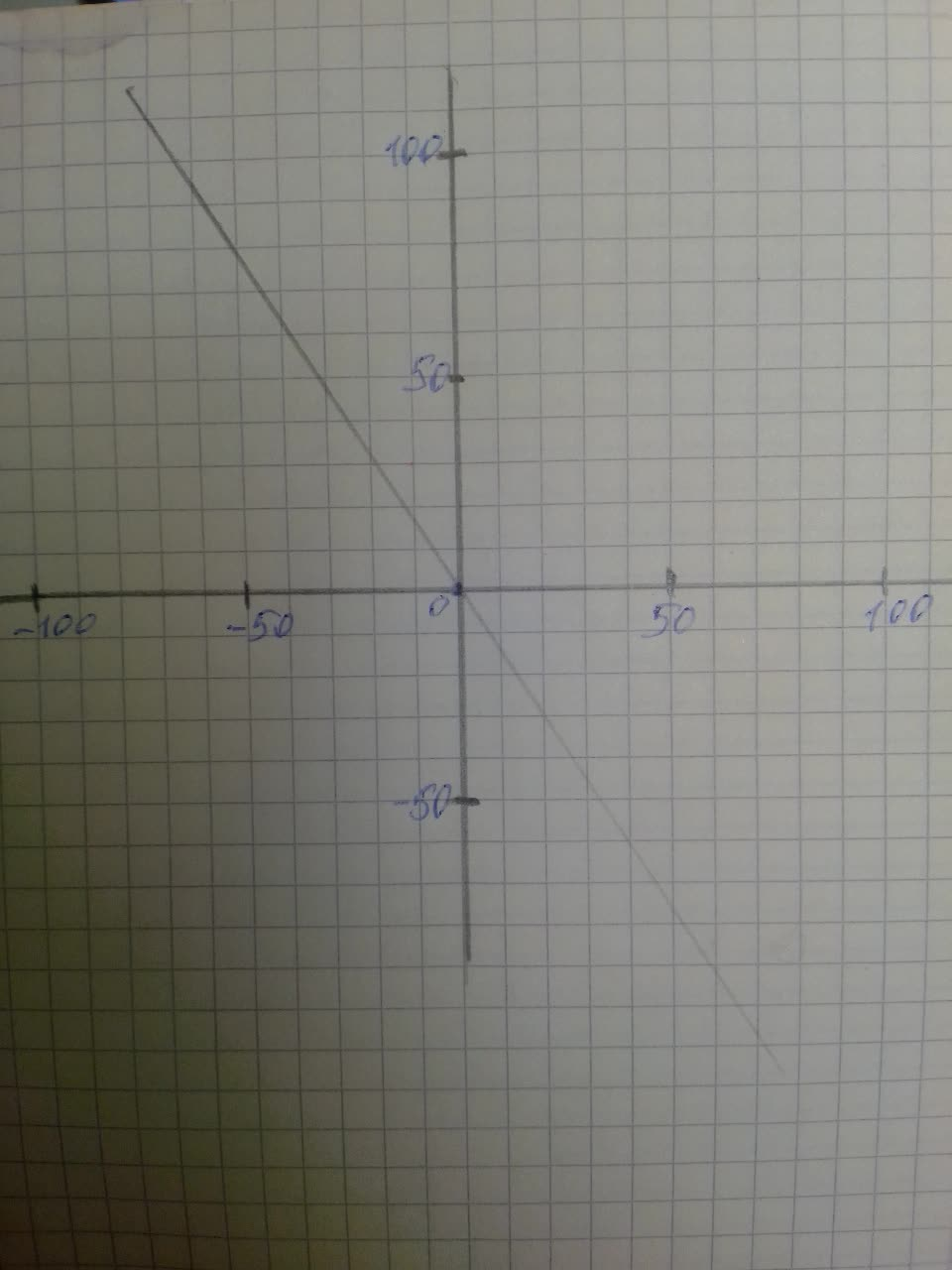# For Exercise, an equation of a degenerate conic section is given. Complete the square and describe the graph of each equation.displaystyle{9}{x}{2}+{4}{y}{2}-{24}{y}+{36}={0}Bergen 2020-12-28 Answered

For Exercise, an equation of a degenerate conic section is given. Complete the square and describe the graph of each equation.
$9{x}^{2}+4{y}^{2}-24y+36=0$

You can still ask an expert for help

• Questions are typically answered in as fast as 30 minutes

Solve your problem for the price of one coffee

• Math expert for every subject
• Pay only if we can solve itSchulzD
Step 1
The given equation is $9{x}^{2}+4{y}^{2}-24y+36=0.$
Use completing the square method and obtain the standard form
Step 2
$4{y}^{2}-24y=-9{x}^{2}-36$
$4\left({y}^{2}-6y\right)=-9{x}^{2}-36$
$4\left({y}^{2}-6y+{3}^{2}-{3}^{2}\right)=-9{x}^{2}-36$
$4{\left(y+3\right)}^{2}-36=-9{x}^{2}-36$
$4{\left(y+3\right)}^{2}=-9{x}^{2}$
${\left(y+3\right)}^{2}=-{\left(\frac{3}{2}x\right)}^{2}$
$y+3=-\frac{3}{2}x$
$2y+6=-3x$
$4x+2y+6=0$
Step 3
Draw the graph of $4x+2y+6=0$